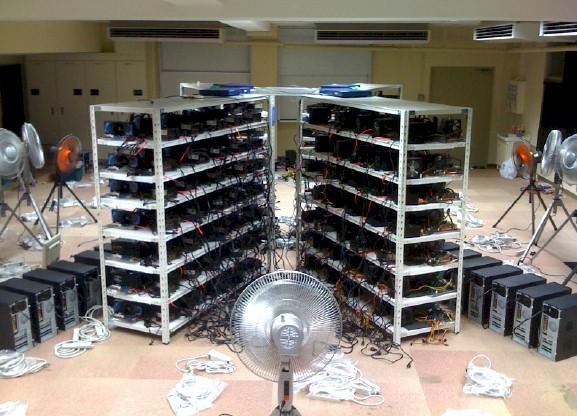## 训练成果，啊这也需要训练？

`````` 1 import scipy.signal as sp
2 import numpy as np
3 # Fix some image dimensions
4 I_width = 100
5 I_height = 70
6 # Generate input image
7 A=np.zeros((I_height,I_width))
8 # Generate random test position
9 pos_x = np.random.randint(0, I_width-1)
10 pos_y = np.random.randint(0, I_height-1)
11 # Put a pixel in a random test position
12 A[pos_y, pos_x]=1
13 # Create what will be the coordinate features
14 X=np.zeros_like(A)
15 Y=np.zeros_like(A)
16 # Fill the X-coordinate value
17 for x in range(I_width):
18    X[:,x] = x
19 # Fill the Y-coordinate value
20 for y in range(I_height):
21    Y[y,:] = y
22 # Define the convolutional operators
23 op1 = np.array([[0, 0, 0],
24                 [0, -1, 0],
25                 [0, 0, 0]])
26 opx = np.array([[0, 0, 0],
27                 [0, I_width, 0],
28                 [0, 0, 0]])
29 opy = np.array([[0, 0, 0],
30                 [0, I_height, 0],
31                 [0, 0, 0]])
32 # Convolve to get the first feature map DY
33 CA0 = sp.convolve2d(A, opy, mode='same')
34 CY0 = sp.convolve2d(Y, op1, mode='same')
35 DY=CA0+CY0
36 # Convolve to get the second feature map DX
37 CA1 = sp.convolve2d(A, opx, mode='same')
38 CX0 = sp.convolve2d(X, op1, mode='same')
39 DX=CA1+CX0
40 # Apply half rectifying nonlinearity
41 DX[np.where(DX<0)]=0
42 DY[np.where(DY<0)]=0
43 # Subtract from a constant (extra layer with a bias unit)
44 result_y=I_height-DY.sum()
45 result_x=I_width-DX.sum()
46 # Check the result
47 assert(pos_x == int(result_x))
48 assert(pos_y == int(result_y))
49 print result_x
50 print result_y``````

## 100个GPU

Appendix里面，有这样一个表格。

Uber团队拿他们的坐标特征，在ImageNet上搞了一下。

## 请开始你的表演

վ'ᴗ' ի 追踪AI技术和产品新动态